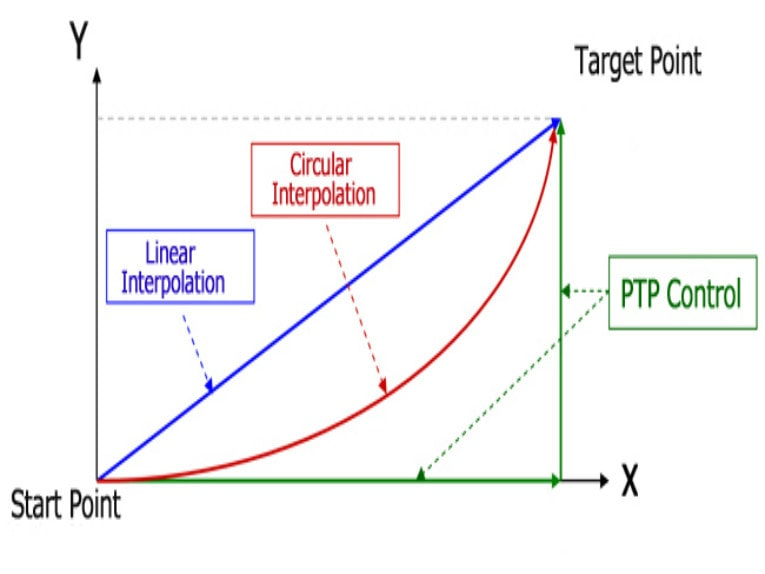# What types of motion can you create with multi-axis linear systems?Point-to-point motion, Blended motion, Contoured motion.

For many tasks, multi-axis linear systems — Cartesian robots, X-Y tables, and gantry systems — travel in straight lines to achieve quick point-to-point movements. But some applications, such as dispensing and cutting, require the system to follow a circular path or a complex shape that can’t be created by simple lines and arcs. Fortunately, modern controllers have the processing power and computing speed to determine and execute complex motion trajectories for multi-axis systems with two, three, or even more axes of motion.

Point-to-point motion

The basic premise of point-to-point motion is to reach a specified point without regard to the path taken. In its simplest form, point-to-point motion moves each axis independently to reach the target position. For example, to move from point (0,0) to point (200, 500), in millimeters, the X axis will move 200 mm, and once it has reached its position, the Y axis will move 500 mm. Moving in two segments independently is typically the slowest method to get from one point to another, so this form of point-to-point motion is rarely used.

The other option for point-to-point motion is to move the axes simultaneously with the same move profile. In the example above — moving from (0,0) to (200, 500) — the X axis would finish its move before the Y axis completed its move, so the motion path would consist of two connected lines.

Blended motion

A variation of point-to-point motion for multi-axis linear systems is blended motion. To create a blended move, the controller overlaps, or blends, the move profiles of two axes. As one axis ends its move, the other axis begins its move, without waiting for the previous axis to fully stop. A user-specified “blend factor” defines the location, time, or velocity value at which the second axis should begin moving.

Blended motion produces a radius, rather than a sharp corner, when the motion changes direction. Applications such as dispensing and cutting may require blended motion if the part or item being tracked has rounded corners. And even if a radius (curve) is not required at the corner of a move, blended motion provides the benefit of keeping the axes moving, avoiding the deceleration and acceleration time required to stop and restart as the motion abruptly changes direction.

Linear interpolation

A more common type of motion for multi-axis systems is linear interpolation, which coordinates motion between the axes. With linear interpolation, the controller determines the appropriate move profile for each axis so that all axes reach the target position at the same time. The result is a straight line — the shortest path — between the start and end points. Linear interpolation can be used for 2- and 3-axis systems.

Circular interpolation

For circular motion paths, or motion along an arc, multi-axis linear systems can use circular interpolation. This motion type works much the same way as linear interpolation, but it requires knowledge of the parameters of the circle, or arc, to be followed, such as center point, radius, and direction, or center point, start angle, direction, and end angle. Circular interpolation takes place in two axes (typically X and Y), but if Z-axis motion is added, the result is helical interpolation.

Contoured motion

Contouring is used when a multi-axis system should follow a specific path to reach the end point, but the trajectory is too complex to define using a series of straight lines and/or arcs. To achieve contoured motion, a series of points is provided during control programming, along with the time for the move, and the motion controller uses linear and circular interpolation to form a continuous path that runs through the points.

A variation of contoured motion, referred to as PVT motion (position, velocity and time), avoids abrupt velocity changes and smooths the trajectories between points by specifying the target velocity (in addition to position and time) at each point.

Post time: Jan-06-2020